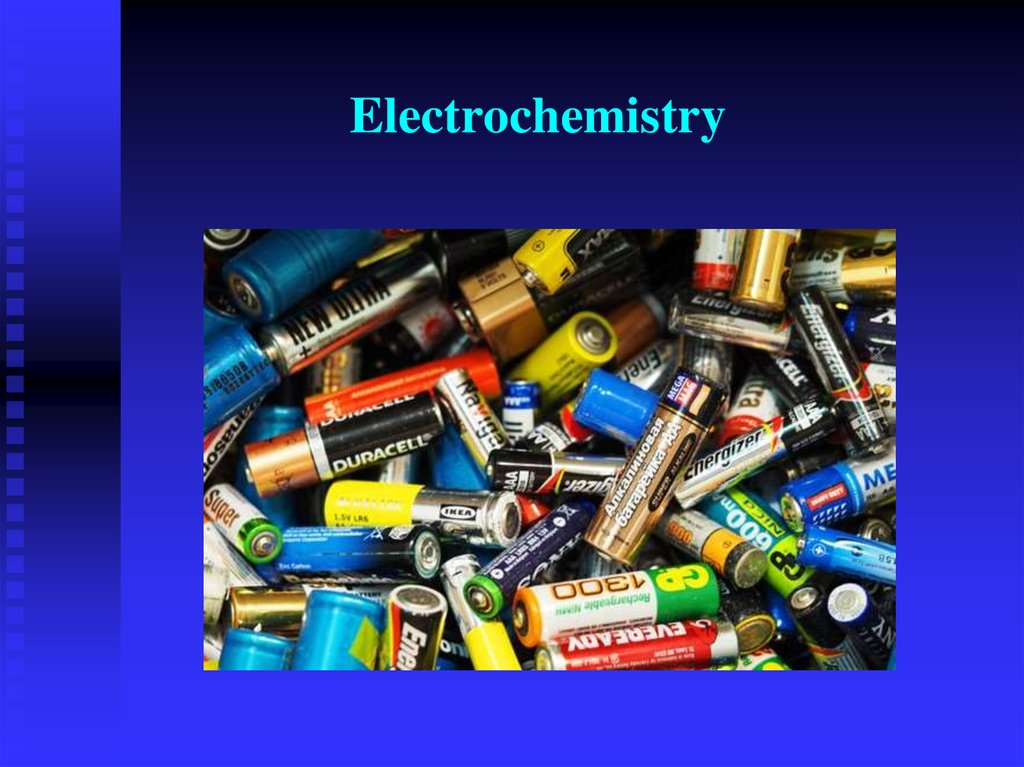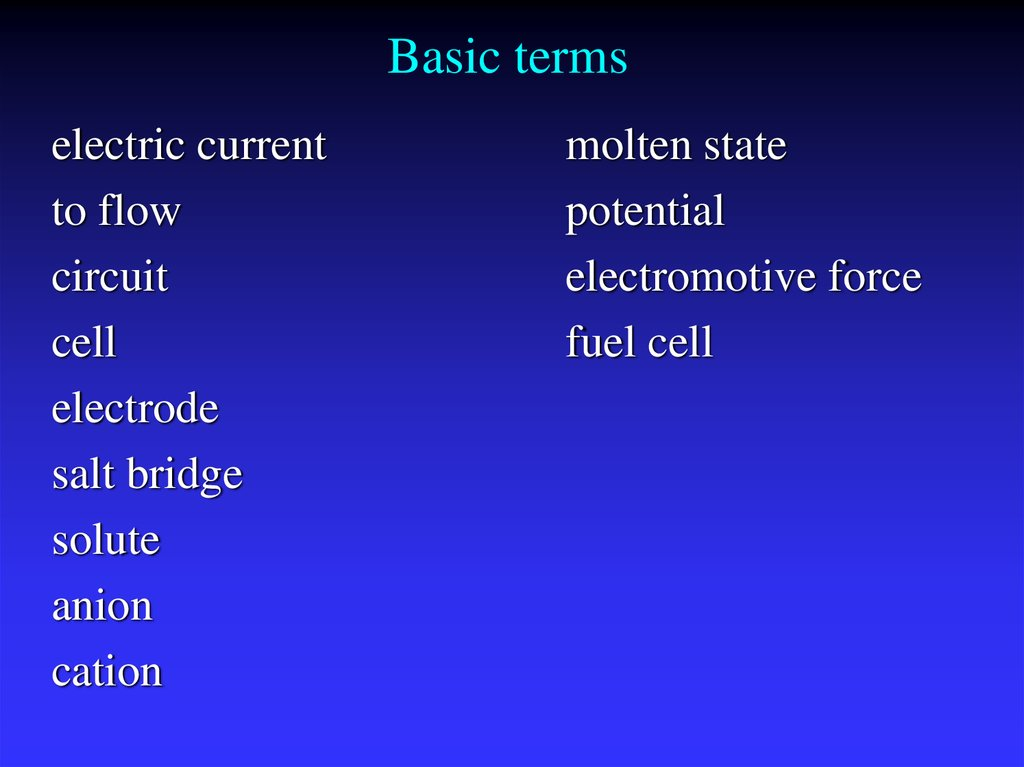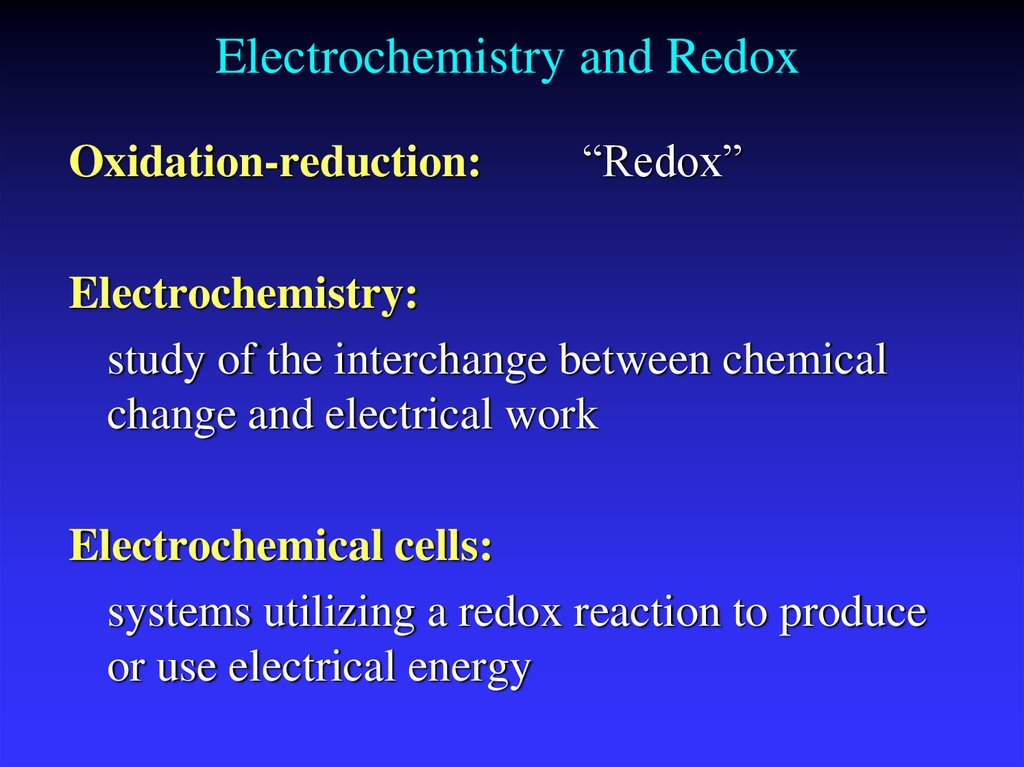# Electrochemistry

## 2. Basic terms

electric current
to flow
circuit
cell
electrode
salt bridge
solute
anion
cation
molten state
potential
electromotive force
fuel cell

## 3. Electrochemistry and Redox

Oxidation-reduction:
“Redox”
Electrochemistry:
study of the interchange between chemical
change and electrical work
Electrochemical cells:
systems utilizing a redox reaction to produce
or use electrical energy

## 4. Redox

Oxidation is loss of eO.N. increases (more positive)
Reduction is gain of eO.N. decreases (more negative)
Oxidation involves loss
Reduction involves gain
OIL
RIG

## 7. Types of cells

Voltaic (galvanic) cells:
a spontaneous reaction generates electrical energy
Chemistry→Electricity
Electrolytic cells:
absorb free energy from an electrical source to drive
a nonspontaneous reaction
Electricity→Chemistry

## 8. Common Components

Electrodes:
conduct electricity between cell and
surroundings
Electrolyte:
mixture of ions involved in reaction or
carrying charge
Salt bridge:
completes circuit (provides charge balance)

## 9. Electrodes

Active electrodes: participate in redox
Inactive: sites of ox. and red.

## 10. Voltaic (Galvanic) Cells

A device in which chemical energy is changed to
electrical energy. Uses a spontaneous reaction.
17_360
e–
e–
e–
e–
Porous disk
e–
(a)
Reducing
agent
Anode
Oxidizing
agent
(b)
e–
Cathode

## 14. Zn2+(aq) + Cu(s)  Cu2+(aq) + Zn(s)

Zn2+(aq) + Cu(s) Cu2+(aq) + Zn(s)
Zn
gives up electrons to Cu
— “pushes harder” on e— greater potential energy
— greater “electrical potential”
Spontaneous reaction due to
— relative difference in metals’ abilities to give e— ability of e- to flow

## 15.

Designing a cell
• half-equations representing reactions in each half-cell
• overall ionic equation
• polarity of electrodes and their nature (anode and
cathode)
• oxidizing agent and reducing agent
• direction of flow of electrons through the conducting
wires and of ions
through the salt bridge
• physical changes occurring at the electrodes or in their
vicinity (metal
deposition, electrode dissolution, changes in pH and so
on).

17_363
e–
e–
Zn(s)
e–
Ecell = +1.10 V
+
Zn 2

SO 4 2
1.0 M Zn 2
solution
Anode
e–
+
Cu 2

SO 4 2
+
+
1.0 M Cu 2
solution
Cathode
Cu(s)

## 18.

Cell Potential
A galvanic cell consists of an oxidizing agent (in
cathode half-cell) and a reducing agent (in anode halfcell).
Electrons flows through a wire from the anode halfcell to the cathode half-cell.
The driving force that allows electrons to flow is
called the electromotive force (emf) or the cell
potential (Ecell).
The unit of electrical potential is volt (V).
1 V = 1 J/C of charge transferred.

## 19. Standard Reduction Potentials

E0 values for reduction half-reactions with
solutes at 1M and gases at 1 atm
Cu2+ + 2e Cu
E0 = 0.34 V vs. SHE
SO42 + 4H+ + 2e H2SO3 + H2O
E0 = 0.20 V vs. SHE

## 22. Calculating E0cell

E0cell = E0cathode - E0anode
E0cell > 0
Spontaneous
E0cell < 0
Not
E0cell = 0
Equilibrium

## 24.

The Nernst equation is an
equation that relates the
reduction potential of an
electrochemical reaction
(half-cell or full cell reaction)
to the standard electrode
potential, temperature, and
activities (often approximated
by concentrations) of the
chemical species undergoing
reduction and oxidation.
Walther Nernst
(1864-1941)

## 25. Nernst Equation

Under nonstandard conditions
G G 0 RTlnQ
nFE nFE RTlnQ
0
E cell
RT
E
lnQ
nF
298K
E cell
0.0592
E
lnQ
n
0
0

## 26.

Ecell is the cell potential (electromotive force) at the
temperature of interest,
Eocell is the standard cell potential,
R is the universal gas constant: R = 8.314472(15) J
K−1 mol−1,
T is the temperature in kelvins
F = 9.64853399(24)×104 C mol−1,
Qr is the reaction quotient of the cell reaction.

## 27. Batteries

A battery is a galvanic cell or, more
commonly, a group of galvanic
cells connected in series.

## 28. Leclanché Acidic Dry Cell

Electrolyte in paste form

ZnCl2 + NH4Cl
Or MgBr2
Anode = Zn (or Mg)
Zn(s) Zn2+(aq) + 2 e−
Cathode = graphite rod
MnO2 is reduced.
2 MnO2(s) + 2 NH4+(aq) + 2 H2O(l) + 2 e−
2 NH4OH(aq) + 2 Mn(O)OH(s)
Cell voltage = 1.5 V
Expensive, nonrechargeable, heavy,
easily corroded

## 29. Alkaline Dry Cell

Same basic cell as acidic dry cell,
except electrolyte is alkaline
KOH paste
Anode = Zn (or Mg)
Zn(s) Zn2+(aq) + 2 e−
Cathode = graphite or brass rod
MnO2 is reduced.
2 MnO2(s) + 2 NH4+(aq) + 2 H2O(l) + 2 e−
2 NH4OH(aq) + 2 Mn(O)OH(s)
Cell voltage = 1.54 V
Longer shelf life than acidic dry cells
and rechargeable, with little
corrosion of zinc.

Six cells in series
Electrolyte = 30% H2SO4
Anode = Pb
Pb(s) + SO42−(aq) PbSO4(s) + 2 e−
Cathode = Pb coated with PbO2
PbO2 is reduced.
PbO2(s) + 4 H+(aq) + SO42−(aq) + 2 e−
PbSO4(s) + 2 H2O(l)
Cell voltage = 2.09 V
Rechargeable, heavy

Electrolyte is concentrated KOH solution
Anode = Cd
Cd(s) + 2 OH−(aq) → Cd(OH)2(s) + 2 e−
E0 = 0.81 V
Cathode = Ni coated with NiO2
NiO2 is reduced.
NiO2(s) + 2 H2O(l) + 2 e− → Ni(OH)2(s) + 2OH−
Cell voltage = 1.30 V
Rechargeable,
long life, light;
however, recharging incorrectly
E0 = 0.49 V

## 32. Ni-MH Battery

Electrolyte is concentrated KOH solution
Anode = metal alloy with dissolved hydrogen

Oxidation of H from H0 to H+
M ∙ H(s) + OH−(aq) → M(s) + H2O(l) + e−
E° = 0.89 V
Cathode = Ni coated with NiO2
NiO2 is reduced.
NiO2(s) + 2 H2O(l) + 2 e− → Ni(OH)2(s) + 2OH−
E0 = 0.49 V
Cell voltage = 1.30 V
Rechargeable, long life, light, more

## 33. Lithium Ion Battery

Electrolyte is concentrated KOH
solution
Anode = graphite impregnated
with Li ions
Cathode = Li - transition
metal oxide
— Reduction of transition metal
Work on Li ion migration from
anode to cathode causing a
corresponding migration of
electrons from anode to cathode
Rechargeable, long life, very light,
more environmentally friendly,
greater energy density

## 35. Fuel Cells

Like batteries in which
reactants are constantly
— So it never runs down!
Anode and cathode both Pt
coated metal
Electrolyte is OH– solution.
Anode reaction
2 H2 + 4 OH– → 4 H2O(l) + 4
e−
Cathode reaction
O2 + 4 H2O + 4 e− → 4 OH–

## 37. Electrolysis

- the process of using electrical
energy to break a compound
apart.
Electrolysis is done in an
electrolytic cell.
Electrolytic cells can be used to
separate elements from their
compounds.

## 40. Electrolytic Cells

The source of energy: a battery or DC power supply.
The positive terminal of the source is attached to the anode.
The negative terminal of the source is attached to the cathode.
Electrolyte can be either an aqueous salt solution or a
molten ionic salt.
Cations in the electrolyte are attracted to the cathode
and anions are attracted to the anode.
Cations pick up electrons from the cathode and are
reduced; anions release electrons to the anode and
are oxidized.

## 44. Michael Faraday (1791- 1867)

1821 - discovered electromagnetic rotation.
1831 - discovered electromagnetic induction, the
principle behind the electricity generator.
1825 - isolated benzene.
1830 - became professor of chemistry at the Royal
1834 - laws of electrolysis